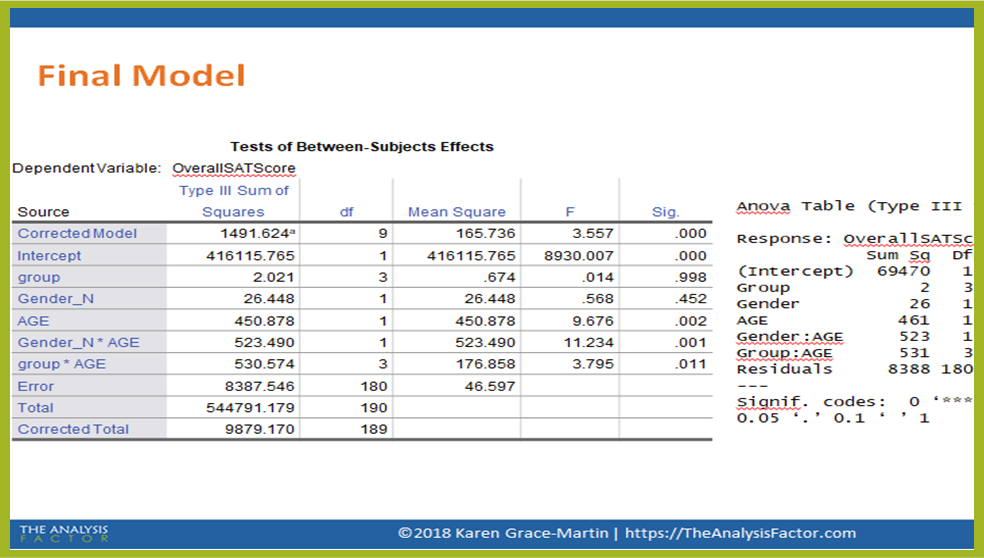Interpreting Linear Regression Coefficients
​​​​​​​A Walk Through Output

Learn how to approach coefficients that are often times ​​​​​​​tricky to interpret.
Instructor: Karen Grace-MartinThere are many coefficients in linear regression models that are difficult to interpret  interactions, categorical predictors, centered predictors. Put them together into one model and it's even harder!

In this webinar recording, join us as we walk our way through actual output and through each of the coefficients so they make sense.​​​​​​​

Covered in this webinar:

1.

Categorical Dummy-Coded Variables

2.

An interaction between the categorical and a continuous variable

3.

Centering

4.

And more!